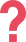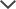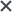I got the Family Membership Plan. I believe that because of these practice tests, my son is now in G&T classes and I've seen my daughter's confidence and scores also improve.
Manju
Just the timing aspect alone helped me mentally prepare for the time constraint. The other major thing that helped me was the math tips study guide.
Shana
I got the ACCUPLACER pack. I found TestPrep-Online’s preparation pack very helpful. The questions and study guides are great. I found that the explanations and solving tips really helped me to learn the material and improve my score.
Nicole
I used the LSAT practice material. It helped improve my technique a lot.
Steve
I got the TEAS practice tests. Your practice test questions are actually very similar to those in the ‘real’ tests.
Helen
We got the NNAT practice pack. The test pack was easy to use and I wouldn't change anything about it. I would definitely recommend this product to other parents.
Michael

## What Is the ACCUPLACER Arithmetic Test?

The ACCUPLACER Arithmetic test is a section of the ACCUPLACER math test, which is part of the college math placement test that also includes an English placement test.
There are 17 questions in this section. These questions evaluate your knowledge across three areas: whole numbers and fractions, decimals and percentages, and applications and problem-solving. The test assesses your knowledge and application of mathematical operations, in which you must solve straightforward mathematical concepts.

## ACCUPLACER Arithmetic Practice Tests

You can practice all the skills needed to perform well on the ACCUPLACER with an ACCUPLACER math practice test. Our developers write ACCUPLACER Arithmetic practice tests to help prepare students for the Arithmetic test. We offer hundreds of math questions and answer explanations in our ACCUPLACER Math Pack. If you are also looking for ACCUPLACER English practice, you can get our ACCUPLACER Premium Pack for a more comprehensive study experience.

## ACCUPLACER Arithmetic Test Topics

Below you will find an outline of the topic material in each of the three sections of the ACCUPLACER Arithmetic test.

### Whole Numbers and Fractions:

• subtraction
• multiplication
• division
• fractions
• estimation

### Decimals and Percentages:

• basic arithmetic using decimals
• recognizing equivalent percentages
• percent problems

### Applications and Problem Solving:

• rate and measurement problems
• fraction problems
• geometry

The ACCUPLACER Arithmetic test, along with the other ACCUPLACER math topics, Elementary Algebra, and College-Level Math, can be daunting. To prepare for the ACCUPLACER, practice math tests as often as you can. You can find plenty of free ACCUPLACER math practice on TestPrep-Online, as well as top-notch practice tests to help you succeed.

## Arithmetic Sample Questions for ACCUPLACER

Below you can find three sample questions for the ACCUPLACER Arithmetic Test. These sample questions include a solution below each question.

Question 1: Level 1 ACCUPLACER Arithmetic Question1.2.3.4.Scroll down to reveal the answer.

First, reduce both fractions to their simplest form. Next, look for the lowest common denominator and then convert the fractions according to this denominator. Finally, add the fractions and, if needed, reduce the fraction.

To simplify, first reduce the fraction by dividing the numerator and denominator by 6:

IMAGE NEEDED

Note that the lowest common denominator is 10.

Rewrite the equation and solve:

IMAGE NEEDED

Reduce the calculated result by dividing the numerator and denominator by 2:

IMAGE NEEDED
The result is:

IMAGE NEEDEDTherefore, the correct answer is (B).

If you chose any other answer, review your calculations. You should look specifically at how to cancel down fractions and add them.

Question 2: Level 2 ACCUPLACER Arithmetic Question

4186 ÷ 0.001 = ?

1. 4,186,000
2. 418,600
3. 41,860
4. 41.86

Scroll down to reveal the answer.

To solve this problem, note that you can move the decimal point an equal number of spaces on each side of the division sign without changing the division value.

4186. ÷ 0.001 =

41860. ÷ 0.01 =

418600. ÷ 0.1 =

4186000. ÷ 1. = 4,186,000

Therefore, the correct answer is (A).

Question 3: Level 3 ACCUPLACER Arithmetic Question

There are 60 monks in the Gate Way Monastery.

20% keep an oath of silence for one day a week. 50% keep an oath of silence for two days a week. 10% keep an oath of silence all week. The rest do not keep an oath of silence at all.

On average, how many monks keep an oath of silence per day?

1. 6
2. 2.7
3. 12
4. 1.9

Scroll down to reveal the answer.

To solve this problem, calculate how many monks, out of the total, keep the silence oath. Then, calculate the average per day.

20% of 60 monks:12 monks keep the oath once a week.

50% of 60 monks:30 monks keep the oath twice a week.

10% of 60 monks:6 monks keep the oath all week.

The rest:12 monks do not keep an oath of silence.

Calculate the average number of monks that the keep the oath per day:Therefore, the correct answer is (D).

If you chose answers (A) or (C), check how to solve average problems.

If you chose answer (B), you probably divided by the number of days per week and not by the number of monks.

## ACCUPLACER Math Study Guide – Free Questions

You can find ACCUPLACER Arithmetic questions in our free ACCUPLACER study guide. You can use these questions and answer explanations to get an idea of the types of questions you will find on both the real ACCUPLACER test and our ACCUPLACER practice packs.

## Start Your ACCUPLACER Arithmetic Practice Now

If you have a math placement test soon, start your practice with us. You can begin preparing for the ACCUPLACER Arithmetic test section with two of our practice packs: the ACCUPLACER Math Pack or the ACCUPLACER Premium Pack. Both packs come with questions, answers, and detailed explanations. Prepare to ace the ACCUPLACER with TestPrep-Online.

ACCUPLACER® and WritePlacer® are trademarks and property of COLLEGE BOARD. The trademark holder is not affiliated with TestPrep-Online or this website.

Need HelpNeed Help